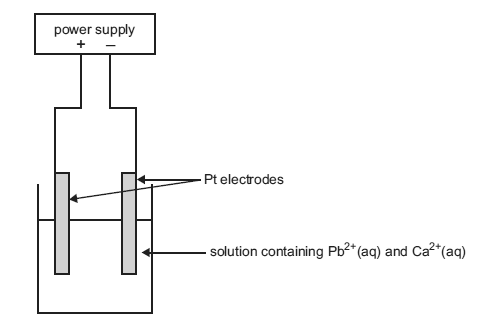Electrolysis 2005 VCE A copper disc is to be silver-plated in an electrolytic cell. The disc forms one electrode and a silver rod the other electrode. The electrolyte provides a source of Ag+(aq). The disc to be plated is connected to the A. positive terminal of a battery so that oxidation occurs at the disc. B. positive terminal of a battery so that reduction occurs at the disc. C. negative terminal of a battery so that oxidation occurs at the disc. D. negative terminal of a battery so that reduction occurs at the disc. Solution The mass of silver to be deposited is 0.150 g. If the current is held steady at 1.50 amps, the time, in seconds, that it takes to complete the plating is closest to A. 90 B. 180 C. 200 D. 360 Solution An identical disc is to be gold-plated with a solution containing Au3+(aq) as the electrolyte using a current of 1.50 amps. The ratio of the time that is needed to plate the disc with 0.150 g of gold to the time needed to plate the disc with 0.150 g of silver is closest to A. 1 to 3 B. 1 to 1.6 C. 1.6 to 1 D. 3 to 1 SolutionA mineral ore contains a mixture of compounds of lead and calcium, in approximately equal proportions. A chemist extracts the metal ions by roasting the ore in air and treating the product with acid. The solution that contains the Pb2+(aq) and Ca2+(aq) is then placed in an electrolytic cell as shown in the diagram below.Label the anode and cathode of the cell. Solution When the current begins to flow in the cell, write equations for the half reaction that is likely to occur at the  positive electrode  negative electrode Solution After some time has elapsed, a new half reaction occurs at one of the electrodes. Write the equation for this half reaction. Solution If the chemist had used copper electrodes instead of platinum electrodes, how would this have affected the half reaction at the anode? Solution